# Electronics and Communication Engineering - Power Electronics

### Exercise :: Power Electronics - Section 2

26.

Which of these commutation methods uses an auxiliary SCR?

 A. Class A B. Class B C. Class C D. Class D

Explanation:

Class C commutation uses an auxiliary SCR and it is also called auxiliary commutation.

27.

A thyristor needs protection against

 A. high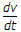B. high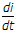C. both highand highD. either highor highExplanation:

Both highand highcan destroy SCR.

28.

Two semiconverters are connected in series to form a single phase series converter. The input v = Vm sin ωt and a1 = a2 are the firing angles of the two converters. If a1 = a2 = 0, the dc output voltage is

 A.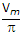B.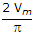C.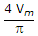D.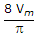Explanation:

Output voltage isfor each.

29.

In integral cycle control the period of on-off control should be more than mechanical time constant of load.

 A. True B. False

Explanation:

It should be less than mechanical time constant.

30.

A single phase half wave converter is feeding a resistive load. Just before triggering, the voltage across thyristor is

 A. zero B. about 1 V C. same as input voltage D. twice the input voltage